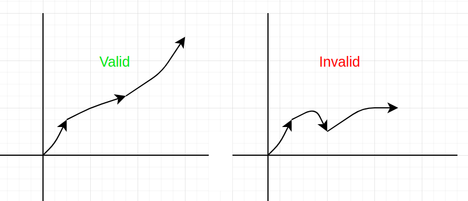# Optimal Walkhumayan7711Intra SUST Programming Co...
Limits 2s, 512 MB

IIKI is in great trouble. In the game called BIIKI, The Hero CIIKI gets power $M$ for per kilometer distance covered in the X-direction and power $N$ for per kilometer distance covered in the Y-direction. Initially, CIIKI is in (0,0) coordinate and can go any direction but not in any negative direction.

More formaly, he can go from (x, y) to (a, b) if and only if $x \leq a\ and\ y \leq b$.You are given the distance $D$ which will be covered by CIIKI.
What is the maximum amount of power CIIKI will get if IIKI plays optimally?

## Input

The first line contains one integer $T$ ( $1\leqslant T \leqslant 5*10^5$ ) — the number of test cases. Each test case is represented by one line containing three integers $M$, $N$, and $D$.

Constraints

• $-10^9\leqslant$ $M, N \leqslant 10^9$
• $0\leqslant$ $D \leqslant 10^9$

## Output

Print T numbers the maximum amount of power CIIKI can achieve.
Your answer will be considered correct if its absolute or relative error doesn't exceed $10^{-6}$.
Formally, if your answer is $a$ and judge's answer is $b$ then your answer will be considered correct if $\frac {|a-b|} {max(1, |b|)} \leq 10^{-6}$.

## Sample

InputOutput
3
5 0 4
-1 2 3
-3 -4 3

20
6
-9


### Submit